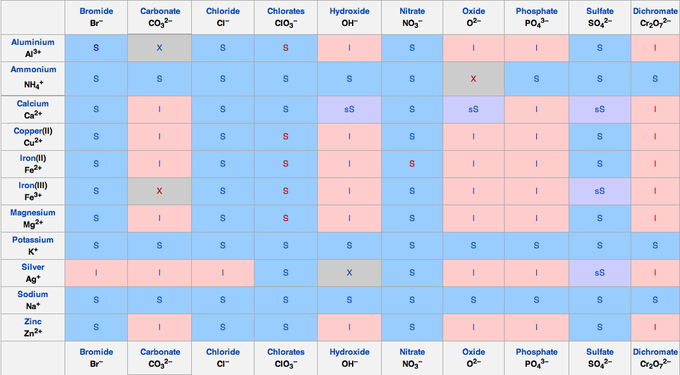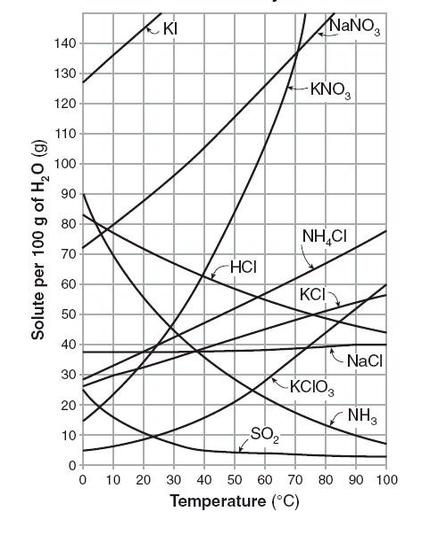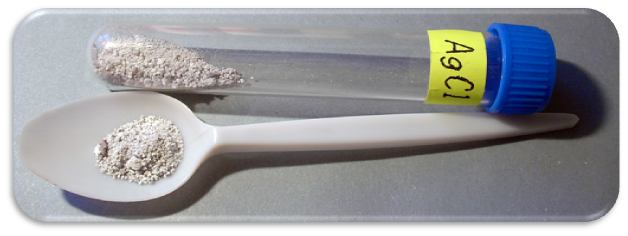## Precipitation Reactions

Precipitation reactions transform ions into an insoluble salt in aqueous solution.

### Learning Objectives

Distinguish ways to write precipitation reactions (complete ionic equation and net ionic equation) and use a solubility table to determine whether a precipitation reaction will occur

### Key Takeaways

#### Key Points

• A precipitation reaction refers to the formation of an insoluble salt when two solutions containing soluble salts are combined. The insoluble salt that falls out of solution is known as the precipitate, hence the reaction’s name.
• Precipitation reactions can help determine the presence of various ions in solution.
• A solubility table can be used to predict precipitation reactions.

#### Key Terms

• precipitation: the process of an insoluble salt forming from its aqueous ions and falling out of solution
• net ionic equation: a method or writing a precipitation reaction without spectator ions

Precipitation refers to a chemical reaction that occurs in aqueous solution when two ions bond together to form an insoluble salt, which is known as the precipitate.

A precipitation reaction can occur when two solutions containing different salts are mixed, and a cation/anion pair in the resulting combined solution forms an insoluble salt; this salt then precipitates out of solution.

The following is a common laboratory example of a precipitation reaction. Aqueous silver nitrate (AgNO3) is added to a solution containing potassium chloride (KCl), and the precipitation of a white solid, silver chloride (AgCl), is observed:

AgNO3 (aq) + KCl (aq) → AgCl (s) + KNO3(aq)

Note that the product silver chloride is the precipitate, and it is designated as a solid. This reaction can be also be written in terms of the individual dissociated ions in the combined solution. This is known as the complete ionic equation:

Ag+ (aq) + NO3(aq) + K+ (aq) + Cl(aq) → AgCl (s) + K+ (aq) + NO3(aq)

A final way to represent a precipitation reaction is known as the net ionic equation. In this case, any spectator ions (those that do not contribute to the precipitation reaction) are left out of the formula completely. Without the spectator ions, the reaction equation simplifies to the following:

Ag+(aq) + Cl(aq) → AgCl (s)

Observing precipitation reactions can be useful in the laboratory to determine the presence of various ions in solution. For instance, if silver nitrate is added to a solution of an unknown salt and a precipitate is observed, the unknown solution might contain chloride (Cl).

Lastly, to make predictions about precipitation reactions, it is important to remember solubility rules. The following solubility chart gives a useful summary:Solubility chart: To determine the solubility of an given salt, find the cationic component along the left-hand side, match it to the anionic component along the top, then check to see if it is S – soluble, I – insoluble, or sS – slightly soluble.

## Solubility

Solubility is the relative ability of a solute (solid, liquid, or gas) to dissolve into a solvent and form a solution.

### Learning Objectives

Recognize the various ions that cause a salt to generally be soluble/insoluble in water.

### Key Takeaways

#### Key Points

• Solubility is the relative ability of a solute to dissolve into a solvent.
• Several factors affect the solubility of a given solute in a given solvent. Temperature often plays the largest role, although pressure can have a significant effect for gases.
• To predict whether a compound will be soluble in a given solvent, remember the saying, “Like dissolves like.” Highly polar ionic compounds such as salt readily dissolve in polar water, but do not readily dissolve in non-polar solutions such as benzene or chloroform.

#### Key Terms

• solute: the compound that dissolves in solution (can be a solid, liquid, or gas)
• solubility: the relative ability of a solute to dissolve into a solvent
• solvent: the compound (usually a liquid) that dissolves the solute

### Definition of Solubility

Solubility is the ability of a solid, liquid, or gaseous chemical substance (referred to as the solute) to dissolve in solvent (usually a liquid) and form a solution. The solubility of a substance fundamentally depends on the solvent used, as well as temperature and pressure. The solubility of a substance in a particular solvent is measured by the concentration of the saturated solution. A solution is considered saturated when adding additional solute no longer increases the concentration of the solution.

The degree of solubility ranges widely depending on the substances, from infinitely soluble (fully miscible), such as ethanol in water, to poorly soluble, such as silver chloride in water. The term “insoluble” is often applied to poorly soluble compounds. Under certain conditions, the equilibrium solubility can be exceeded, yielding a supersaturated solution.

Solubility does not depend on particle size; given enough time, even large particles will eventually dissolve.

### Temperature

The solubility of a given solute in a given solvent typically depends on temperature. For many solids dissolved in liquid water, solubility tends to correspond with increasing temperature. As water molecules heat up, they vibrate more quickly and are better able to interact with and break apart the solute.Solubilty of various substances vs. temperature change: Solubility increases with temperature for most substances; for example, more sugar will dissolve in hot water than in cold water.

The solubility of gases displays the opposite relationship with temperature; that is, as temperature increases, gas solubility tends to decrease. In a chart of solubility vs. temperature, notice how solubility tends to increase with increasing temperature for the salts and decrease with increasing temperature for the gases.

### Pressure

Pressure has a negligible effect on the solubility of solid and liquid solutes, but it has a strong effect on solutions with gaseous solutes. This is apparent every time you open a soda can; the hissing sound from the can is due to the fact that its contents are under pressure, which ensures that the soda stays carbonated (that is to say, that the carbon dioxide stays dissolved in solution). The takeaway from this is that the solubility of gases tends to correlate with increasing pressure.

### Polarity

A popular saying used for predicting solubility is “Like dissolves like.” This statement indicates that a solute will dissolve best in a solvent that has a similar chemical structure; the ability for a solvent to dissolve various compounds depends primarily on its polarity. For example, a polar solute such as sugar is very soluble in polar water, less soluble in moderately polar methanol, and practically insoluble in non-polar solvents such as benzene. In contrast, a non-polar solute such as naphthalene is insoluble in water, moderately soluble in methanol, and highly soluble in benzene.

### Solubility Chart

The solubility chart shows the solubility of many salts. Salts of alkali metals (and ammonium), as well as those of nitrate and acetate, are always soluble. Carbonates, hydroxides, sulfates, phosphates, and heavy metal salts are often insoluble.Solubility chart: The solubilities of salts formed from cations on the left and anions on the top are designated as: soluble (S), insoluble (I), or slightly soluble (sS).

Solubility: Solubility of salt and gas solutes in liquid solvent.

## Molecular, Ionic, and Complete Ionic Equations

Precipitation reactions can be written as molecular, ionic, or complete ionic equations.

### Learning Objectives

Recognize whether a chemical equation is written in molecular, ionic, or complete ionic form.

### Key Takeaways

#### Key Points

• Chemical reactions that proceed through ionic forms can be written in a variety of ways.
• Molecular equations show species reacting as their molecular formula, with subscripts added to indicate their solid, liquid, gaseous, or aqueous nature.
• Ionic equations show species reacting as their ionic components. Subscripts are not needed to describe the state of the matter, because all ions are in aqueous solution. A net ionic equation is one in which spectator ions are removed.
• Spectator ions are present in solution but do not participate in the actual precipitation reaction.

#### Key Terms

• electrolyte: a substance that, when dissolved in solution, will enable the solution to conduct electricity
• salt: An ionic compound that is composed of cations and anions. The constituent ions are held together by ionic bonds, not covalent bonds
• spectator ion: an ion that is present in solution but does not participate in a precipitation reaction

### Molecular Equation

There are various ways to write out precipitation reactions. In the molecular equation, electrolytes are written as salts followed by (aq) to indicate that the electrolytes are completely dissociated into their constituent ions; the (aq) designation indicates that the ions are in aqueous solution. For example, aqueous calcium chloride’s reaction with aqueous silver nitrate can be written as follows:

$\text{CaCl}_{2} (aq)+2\text{AgNO}_{3} (aq) \rightleftharpoons \text{Ca}(\text{NO}_{3})_{2} (aq) + 2\text{AgCl}(s)$

On the right hand side of the equation, the precipitant (AgCl) is written in its full formula and designated as a solid, since this is the precipitate that is formed in the reaction. Note that the remaining salt, Ca(NO3)2, is still designed with (aq) to indicate that the ions are dissociated in solution.

### Complete Ionic and Net Ionic Equations

Because the reactants and one of the products are strong electrolytes, it is possible to write them out in terms of their constituent ions. The resulting equation is known as the complete ionic equation, and it looks as follows:

$\text{Ca}^{2+}(aq) + 2\text{Cl}^{-}(aq) + 2\text{Ag}{+}(aq) + 2\text{NO}_{3}^{-}(aq) \rightarrow \text{Ca}^{2+}(aq) + 2\text{NO}_{3}^{-}(aq) + 2\text{AgCl}(s)$

In this equation, every ion is written out on both sides. The equation is balanced with the molar amount of each ion preceding it. This can be simplified to the net or complete ionic equation, which is shown below:

$2\text{Cl}^{-}(aq) + 2\text{Ag}^{+}(aq) \rightarrow 2\text{AgCl} (s)$

In this representation, the ions that are uninvolved in the precipitation reaction ($\text{Ca}^{2+}$ and $\text{NO}_{3}^{-}$) are simply excluded from the reaction, because they are essentially redundant on both the left and right side. In this particular instance, the equation can be further simplified to the reduced balanced form, with the cation coming before the anion as is most commonly practiced:

$\text{Ag}^{+}(aq) + \text{Cl}^{-}(aq) \rightarrow \text{AgCl} (s)$

The precipitated silver chloride appears as a white solid.Silver chloride: Silver chloride is a precipitant of silver and chloride ions reacting in solution.

The $\text{Ca}^{2+}\text{NO}_{3}^{-}$and the ions remain in solution and are not part of the reaction. They are termed spectator ions because they do not participate directly in the reaction; rather, they exist with the same oxidation state on both the reactant and product side of the chemical equation. They are only needed for charge balance of the original reagents.